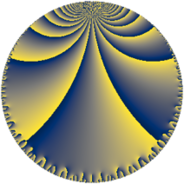# Properties

 Label 76.7.jLevel $76$ Weight $7$ Character orbit 76.j Rep. character $\chi_{76}(13,\cdot)$ Character field $\Q(\zeta_{18})$ Dimension $60$ Newform subspaces $1$ Sturm bound $70$ Trace bound $0$

# Related objects

## Defining parameters

 Level: $$N$$ $$=$$ $$76 = 2^{2} \cdot 19$$ Weight: $$k$$ $$=$$ $$7$$ Character orbit: $$[\chi]$$ $$=$$ 76.j (of order $$18$$ and degree $$6$$) Character conductor: $$\operatorname{cond}(\chi)$$ $$=$$ $$19$$ Character field: $$\Q(\zeta_{18})$$ Newform subspaces: $$1$$ Sturm bound: $$70$$ Trace bound: $$0$$

## Dimensions

The following table gives the dimensions of various subspaces of $$M_{7}(76, [\chi])$$.

Total New Old
Modular forms 378 60 318
Cusp forms 342 60 282
Eisenstein series 36 0 36

## Trace form

 $$60q + 30q^{3} - 216q^{7} + 690q^{9} + O(q^{10})$$ $$60q + 30q^{3} - 216q^{7} + 690q^{9} + 1680q^{11} - 2940q^{13} + 2496q^{15} - 5112q^{17} - 15792q^{19} - 32076q^{21} - 19272q^{23} + 58896q^{25} - 124830q^{27} + 126840q^{29} + 30780q^{31} - 274470q^{33} - 226284q^{35} + 178968q^{39} + 83394q^{41} + 418848q^{43} - 95472q^{45} - 498696q^{47} - 744330q^{49} + 1334538q^{51} + 458004q^{53} - 260136q^{55} - 981984q^{57} - 523362q^{59} - 644172q^{61} + 926832q^{63} + 1337220q^{65} + 1719114q^{67} + 1333800q^{69} - 1895220q^{71} - 1189704q^{73} + 1337256q^{77} + 147432q^{79} + 272130q^{81} + 442800q^{83} + 1479096q^{85} + 1300572q^{87} - 301596q^{89} + 661008q^{91} + 3576q^{93} - 5709984q^{95} + 1386630q^{97} + 3822798q^{99} + O(q^{100})$$

## Decomposition of $$S_{7}^{\mathrm{new}}(76, [\chi])$$ into newform subspaces

Label Dim. $$A$$ Field CM Traces $q$-expansion
$$a_2$$ $$a_3$$ $$a_5$$ $$a_7$$
76.7.j.a $$60$$ $$17.484$$ None $$0$$ $$30$$ $$0$$ $$-216$$

## Decomposition of $$S_{7}^{\mathrm{old}}(76, [\chi])$$ into lower level spaces

$$S_{7}^{\mathrm{old}}(76, [\chi]) \cong$$ $$S_{7}^{\mathrm{new}}(19, [\chi])$$$$^{\oplus 3}$$$$\oplus$$$$S_{7}^{\mathrm{new}}(38, [\chi])$$$$^{\oplus 2}$$